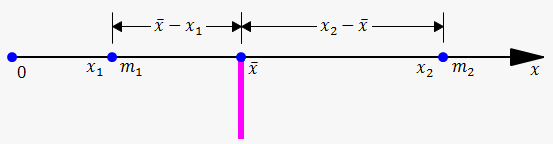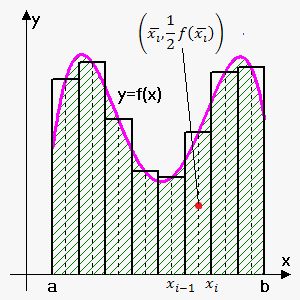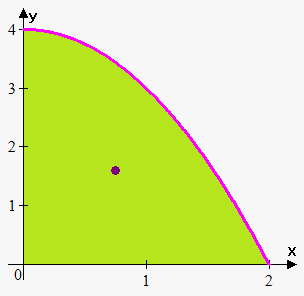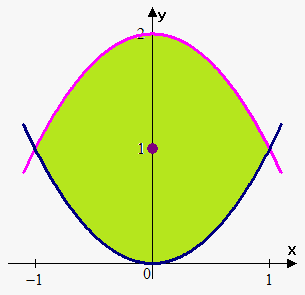Moments and Centers of Mass

In this note we will try to find a point P on which a thin plate of any given shape balances horizontally. This point is called the center of mass (or center of gravity) of the plate.

We first consider the simpler situation. Suppose that the rod lies along the x-axis with ${m}_{{1}}$ at ${x}_{{1}}$ and ${m}_{{2}}$ at ${x}_{{2}}$ and the center of mass at ${\overline{{x}}}$. According to the Law of Lever We see${m}_{{1}}{\left({\overline{{x}}}-{x}_{{1}}\right)}={m}_{{2}}{\left({x}_{{2}}-{\overline{{x}}}\right)}$ or ${\overline{{x}}}=\frac{{{m}_{{1}}{x}_{{1}}+{m}_{{2}}{x}_{{2}}}}{{{m}_{{1}}+{m}_{{2}}}}$.

The numbers ${m}_{{1}}{x}_{{1}}$ and ${m}_{{2}}{x}_{{2}}$ are called the moments of the masses ${m}_{{1}}$ and ${m}_{{2}}$ (with respect to the origin). Above equation says that centers of mass is obtained by adding the moments of masses and dividing by total mass.

In general, if we have a system of ${n}$ particles with masses ${m}_{{1}},{m}_{{2}},\ldots,{m}_{{n}}$ located at the points ${x}_{{1}},{x}_{{2}},\ldots,{x}_{{n}}$ on the x-axis, it can be shown similarly that the center of mass of the system is located at ${\overline{{x}}}=\frac{{{\sum_{{{i}={1}}}^{{n}}}{m}_{{i}}{x}_{{i}}}}{{{\sum_{{{i}={1}}}^{{n}}}{m}_{{i}}}}$.

Sum of the individual moments ${M}={\sum_{{{i}={1}}}^{{n}}}{m}_{{i}}{x}_{{i}}$ is called the moment of the system with respect to the origin and ${m}={\sum_{{{i}={1}}}^{{n}}}{m}_{{i}}$ is the total mass of the system.

Now we consider a system of particles with masses ${m}_{{1}},{m}_{{2}},\ldots,{m}_{{n}}$ located at the points ${\left({x}_{{1}},{y}_{{1}}\right)},{\left({x}_{{2}},{y}_{{2}}\right)},\ldots,{\left({x}_{{n}},{y}_{{n}}\right)}$ in the xy-plane. By analogy with the one-dimensional case, we define the moment of the system about the y-axis to be ${M}_{{y}}={\sum_{{{i}={1}}}^{{n}}}{m}_{{i}}{x}_{{i}}$ and the moment of the system about x-axis as ${M}_{{x}}={\sum_{{{i}={1}}}^{{n}}}{m}_{{i}}{y}_{{i}}$.

${M}_{{y}}$ measures the tendency of the system to rotate about the y-axis and ${M}_{{x}}$ measures the tendency to rotate about the x-axis.
As in the one-dimensional case, the coordinates of the center of mass are given in terms of the moments by the formulas ${\overline{{x}}}=\frac{{{M}_{{y}}}}{{m}}$ and ${\overline{{y}}}=\frac{{{M}_{{x}}}}{{m}}$ where ${m}={\sum_{{{i}={1}}}^{{n}}}{m}_{{i}}$ is the total mass. Since ${m}{\overline{{x}}}={M}_{{y}}$ and ${m}{\overline{{y}}}={M}_{{x}}$, the center of mass ${\left({\overline{{x}}},{\overline{{y}}}\right)}$ is the point where a single particle of mass ${m}$ would have the same moments as the system.

Example 1. Find the moments and center of mass of the system of objects that have masses 5, 7, and 8 at the points (2,1), (3,-5), and (-4,4).

${M}_{{y}}={5}\cdot{2}+{7}\cdot{3}+{8}\cdot{\left(-{4}\right)}=-{1}$.

${M}_{{x}}={5}\cdot{1}+{7}\cdot{\left(-{5}\right)}+{8}\cdot{4}={2}$.

Since ${m}={5}+{7}+{8}={20}$ then ${\overline{{x}}}=\frac{{{M}_{{y}}}}{{m}}=-\frac{{1}}{{20}}$ and ${\overline{{y}}}=\frac{{{M}_{{x}}}}{{m}}=\frac{{2}}{{20}}=\frac{{1}}{{10}}$.

So, center of mass is located at point ${\left(-\frac{{1}}{{20}},\frac{{1}}{{10}}\right)}$.Next we consider a flat plate (called a lamina) with uniform density $\rho$ that occupies a region ${R}$ under continuous curve ${y}={f{{\left({x}\right)}}}$, above x-axis and between lines ${x}={a}$ and ${x}={b}$. We
wish to find the center of mass of the plate, which is called the centroid of ${R}$. In doing this we use the following simple fact: centroid of a rectangle is its center.

As always we divide the interval ${\left[{a},{b}\right]}$ into ${n}$ subintervals with endpoints ${x}_{{0}},{x}_{{1}},\ldots,{x}_{{n}}$ and equal width $\Delta{x}$. We choose the sample point ${{x}_{{i}}^{{\star}}}$ to be the midpoint ${\overline{{{x}_{{i}}}}}$ of the i-th subinterval, that is, ${\overline{{{x}_{{i}}}}}=\frac{{1}}{{2}}{\left({x}_{{{i}-{1}}}+{x}_{{i}}\right)}$.

The centroid of the i-th approximating rectangle ${R}_{{i}}$ is its center ${C}_{{i}}={\left({\overline{{{x}_{{i}}}}},\frac{{1}}{{2}}{f{{\left({\overline{{{x}_{{i}}}}}\right)}}}\right)}$. Its area is ${f{{\left({\overline{{{x}_{{i}}}}}\right)}}}\Delta{x}$, so its mass is $\rho{f{{\left({\overline{{{x}_{{i}}}}}\right)}}}\Delta{x}$.

The moment of ${R}_{{i}}$ about the y-axis is the product of its mass and the distance from ${C}_{{i}}$ to the y-axis, which is ${\overline{{{x}_{{i}}}}}$. Thus, ${M}_{{y}}{\left({R}_{{i}}\right)}={\left(\rho{f{{\left({\overline{{{x}_{{i}}}}}\right)}}}\Delta{x}\right)}{\overline{{{x}_{{i}}}}}=\rho{\overline{{{x}_{{i}}}}}{f{{\left({\overline{{{x}_{{i}}}}}\right)}}}\Delta{x}$. Adding these moments, we obtain the moment of the polygonal approximation to ${R}$, and then by taking the limit as ${n}\to\infty$ we obtain the moment of ${R}$ itself about the y-axis: ${M}_{{y}}=\lim_{{{n}\to\infty}}{\sum_{{{i}={1}}}^{{n}}}\rho{\overline{{{x}_{{i}}}}}{f{{\left({\overline{{{x}_{{i}}}}}\right)}}}\Delta{x}=\rho{\int_{{a}}^{{b}}}{x}{f{{\left({x}\right)}}}{d}{x}$.

Similarly we compute the moment of ${R}_{{i}}$ about the x-axis as the product of its mass and the distance from ${C}_{{i}}$ to the x-axis: ${M}_{{x}}{\left({R}_{{i}}\right)}={\left(\rho{f{{\left({\overline{{{x}_{{i}}}}}\right)}}}\Delta{x}\right)}\frac{{1}}{{2}}{f{{\left({\overline{{{x}_{{i}}}}}\right)}}}=\frac{{1}}{{2}}\rho{{\left({f{{\left({\overline{{{x}_{{i}}}}}\right)}}}\right)}}^{{2}}\Delta{x}$.
Again we add these moments and take the limit to obtain the moment of ${R}$ about the x-axis:

${M}_{{x}}=\lim_{{{n}\to\infty}}{\sum_{{{i}={1}}}^{{n}}}\frac{{1}}{{2}}\rho{{\left({f{{\left({\overline{{{x}_{{i}}}}}\right)}}}\right)}}^{{2}}\Delta{x}=\rho{\int_{{a}}^{{b}}}\frac{{1}}{{2}}{{\left({f{{\left({x}\right)}}}\right)}}^{{2}}{d}{x}$.

Just as for systems of particles, the center of mass of the plate is defined so that ${m}{\overline{{{x}}}}={M}_{{y}}$ and ${m}{\overline{{{y}}}}={M}_{{x}}$. But the mass of the plate is the product of its density and its area: ${m}=\rho{A}=\rho{\int_{{a}}^{{b}}}{f{{\left({x}\right)}}}{d}{x}$.

Therefore,

${\overline{{{x}}}}=\frac{{{M}_{{y}}}}{{m}}=\frac{{\rho{\int_{{a}}^{{b}}}{x}{f{{\left({x}\right)}}}{d}{x}}}{{\rho{\int_{{a}}^{{b}}}{f{{\left({x}\right)}}}{d}{x}}}=\frac{{{\int_{{a}}^{{b}}}{x}{f{{\left({x}\right)}}}{d}{x}}}{{{\int_{{a}}^{{b}}}{f{{\left({x}\right)}}}{d}{x}}}$ and ${\overline{{{y}}}}=\frac{{{M}_{{x}}}}{{m}}=\frac{{\rho{\int_{{a}}^{{b}}}\frac{{1}}{{2}}{{\left({f{{\left({x}\right)}}}\right)}}^{{2}}{d}{x}}}{{\rho{\int_{{a}}^{{b}}}{f{{\left({x}\right)}}}{d}{x}}}=\frac{{{\int_{{a}}^{{b}}}\frac{{1}}{{2}}{{\left({f{{\left({x}\right)}}}\right)}}^{{2}}{d}{x}}}{{{\int_{{a}}^{{b}}}{f{{\left({x}\right)}}}{d}{x}}}$.

Note, that center of mass doesn't depend on density.

The center of mass of the plate (or the centroid of ${R}$) is located at the point ${\left({\overline{{{x}}}},{\overline{{{y}}}}\right)}$, where

${\overline{{x}}}=\frac{{1}}{{A}}{\int_{{a}}^{{b}}}{x}{f{{\left({x}\right)}}}{d}{x}=\frac{{{\int_{{a}}^{{b}}}{x}{f{{\left({x}\right)}}}{d}{x}}}{{{\int_{{a}}^{{b}}}{f{{\left({x}\right)}}}{d}{x}}}$

${\overline{{y}}}=\frac{{1}}{{A}}{\int_{{a}}^{{b}}}\frac{{1}}{{2}}{{\left({f{{\left({x}\right)}}}\right)}}^{{2}}{d}{x}=\frac{{{\int_{{a}}^{{b}}}\frac{{1}}{{2}}{{\left({f{{\left({x}\right)}}}\right)}}^{{2}}{d}{x}}}{{{\int_{{a}}^{{b}}}{f{{\left({x}\right)}}}{d}{x}}}$.

Example 2. Find the center of mass of region bounded by ${y}={4}-{{x}}^{{2}}$ for ${x}\ge{0}$.First let's find area: ${A}={\int_{{0}}^{{2}}}{\left({4}-{{x}}^{{2}}\right)}{d}{x}={\left({4}{x}-\frac{{1}}{{3}}{{x}}^{{3}}\right)}{{\mid}_{{0}}^{{2}}}={4}\cdot{2}-\frac{{1}}{{3}}\cdot{8}=\frac{{16}}{{3}}$.

Now, ${\overline{{{y}}}}=\frac{{1}}{{A}}{\int_{{0}}^{{2}}}\frac{{1}}{{2}}{{\left({4}-{{x}}^{{2}}\right)}}^{{2}}{d}{x}=\frac{{3}}{{{32}}}{\int_{{0}}^{{2}}}{\left({16}-{8}{{x}}^{{2}}+{{x}}^{{4}}\right)}{d}{x}=$

$=\frac{{3}}{{32}}{\left({16}{x}-\frac{{8}}{{3}}{{x}}^{{3}}+\frac{{1}}{{5}}{{x}}^{{5}}\right)}{{\mid}_{{0}}^{{2}}}=\frac{{3}}{{32}}\cdot\frac{{256}}{{15}}=\frac{{8}}{{5}}$.

${\overline{{{x}}}}=\frac{{1}}{{A}}{\int_{{0}}^{{2}}}{x}{\left({4}-{{x}}^{{2}}\right)}{d}{x}=\frac{{3}}{{{16}}}{\int_{{0}}^{{2}}}{\left({4}{x}-{{x}}^{{3}}\right)}{d}{x}=$

$=\frac{{3}}{{16}}{\left({2}{{x}}^{{2}}-\frac{{1}}{{4}}{{x}}^{{4}}\right)}{{\mid}_{{0}}^{{2}}}=\frac{{3}}{{16}}\cdot{4}=\frac{{3}}{{4}}$.

So, center of mass is ${\left(\frac{{3}}{{4}},\frac{{8}}{{5}}\right)}$.

In a similar way if region ${R}$ is bounded by curves ${f{{\left({x}\right)}}}$ and ${g{{\left({x}\right)}}}$ on interval ${\left[{a},{b}\right]}$ and ${f{{\left({x}\right)}}}\ge{g{{\left({x}\right)}}}$ for ${a}\le{x}\le{b}$ then

The center of mass of the plate (or the centroid of ${R}$) is located at the point ${\left({\overline{{{x}}}},{\overline{{{y}}}}\right)}$, where

${\overline{{x}}}=\frac{{1}}{{A}}{\int_{{a}}^{{b}}}{x}{\left({f{{\left({x}\right)}}}-{g{{\left({x}\right)}}}\right)}{d}{x}=\frac{{{\int_{{a}}^{{b}}}{x}{\left({f{{\left({x}\right)}}}-{g{{\left({x}\right)}}}\right)}{d}{x}}}{{{\int_{{a}}^{{b}}}{\left({f{{\left({x}\right)}}}-{g{{\left({x}\right)}}}\right)}{d}{x}}}$

${\overline{{y}}}=\frac{{1}}{{A}}{\int_{{a}}^{{b}}}\frac{{1}}{{2}}{\left({{\left({f{{\left({x}\right)}}}\right)}}^{{2}}-{{\left({g{{\left({x}\right)}}}\right)}}^{{2}}\right)}{d}{x}=\frac{{{\int_{{a}}^{{b}}}\frac{{1}}{{2}}{\left({{\left({f{{\left({x}\right)}}}\right)}}^{{2}}-{{\left({g{{\left({x}\right)}}}\right)}}^{{2}}\right)}{d}{x}}}{{{\int_{{a}}^{{b}}}{\left({f{{\left({x}\right)}}}-{g{{\left({x}\right)}}}\right)}{d}{x}}}$.

Example 3. Find center of mass of the region bounded by ${y}={{x}}^{{2}}$ and ${y}={2}-{{x}}^{{2}}$.First we sketch graph and see that bounds of integration are -1 and 1.

Next, since region is symmetric about x-axis then ${\overline{{{x}}}}={0}$.

${A}={\int_{{-{1}}}^{{1}}}{\left({2}-{{x}}^{{2}}-{{x}}^{{2}}\right)}{d}{x}={\left({2}{x}-\frac{{2}}{{3}}{{x}}^{{3}}\right)}{{\mid}_{{-{1}}}^{{1}}}=\frac{{8}}{{3}}$.

${\overline{{{y}}}}=\frac{{1}}{{A}}{\int_{{-{1}}}^{{1}}}\frac{{1}}{{2}}{\left({{\left({2}-{{x}}^{{2}}\right)}}^{{2}}-{{\left({{x}}^{{2}}\right)}}^{{2}}\right)}{d}{x}=\frac{{3}}{{16}}{\int_{{-{1}}}^{{1}}}{\left({4}-{4}{{x}}^{{2}}\right)}{d}{x}=$

$=\frac{{3}}{{16}}{\left({4}{x}-\frac{{1}}{{3}}{{x}}^{{3}}\right)}{{\mid}_{{-{1}}}^{{1}}}=\frac{{3}}{{16}}\cdot\frac{{16}}{{3}}$.

So, center of mass is ${\left({0},{1}\right)}$. Alternatively we could notice that region is symmetric about line ${y}={1}$, so ${\overline{{{y}}}}={1}$.